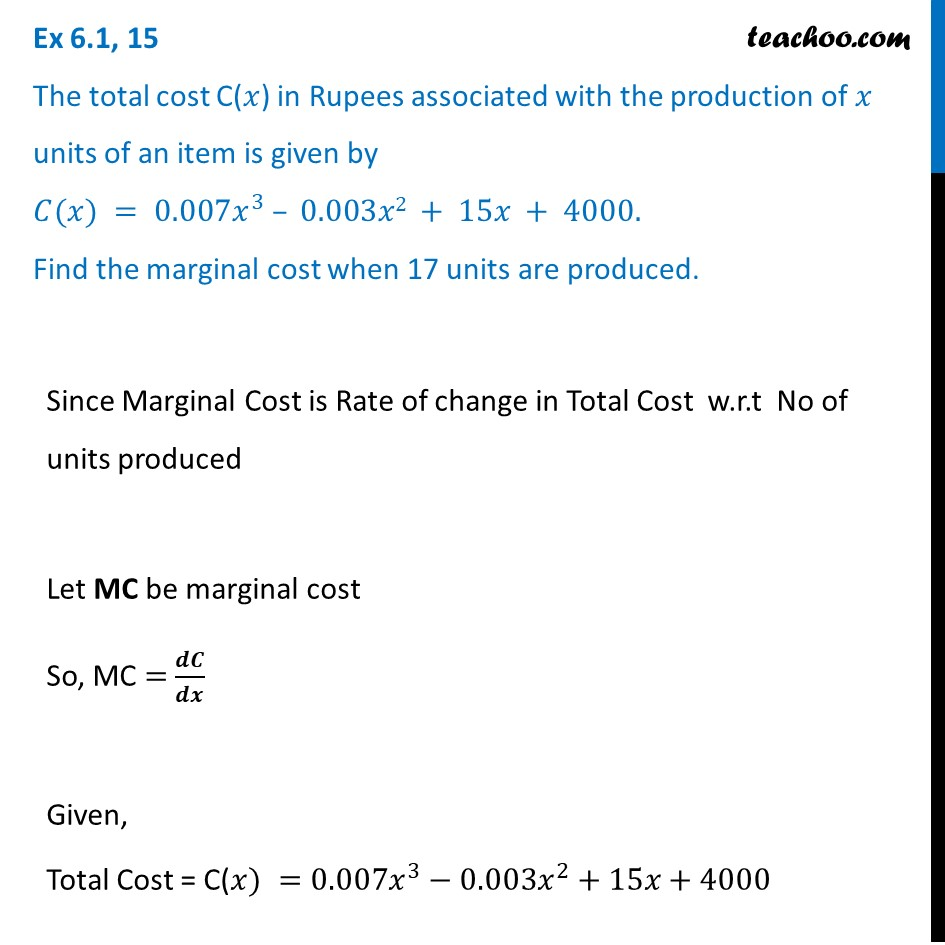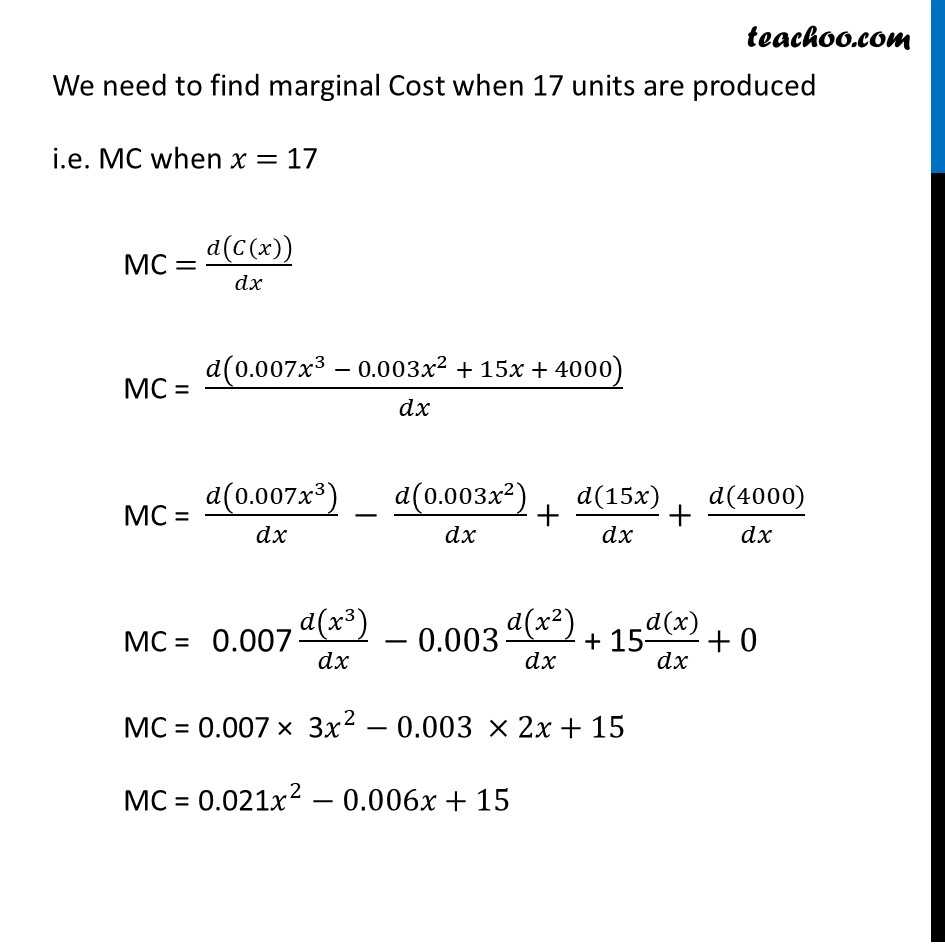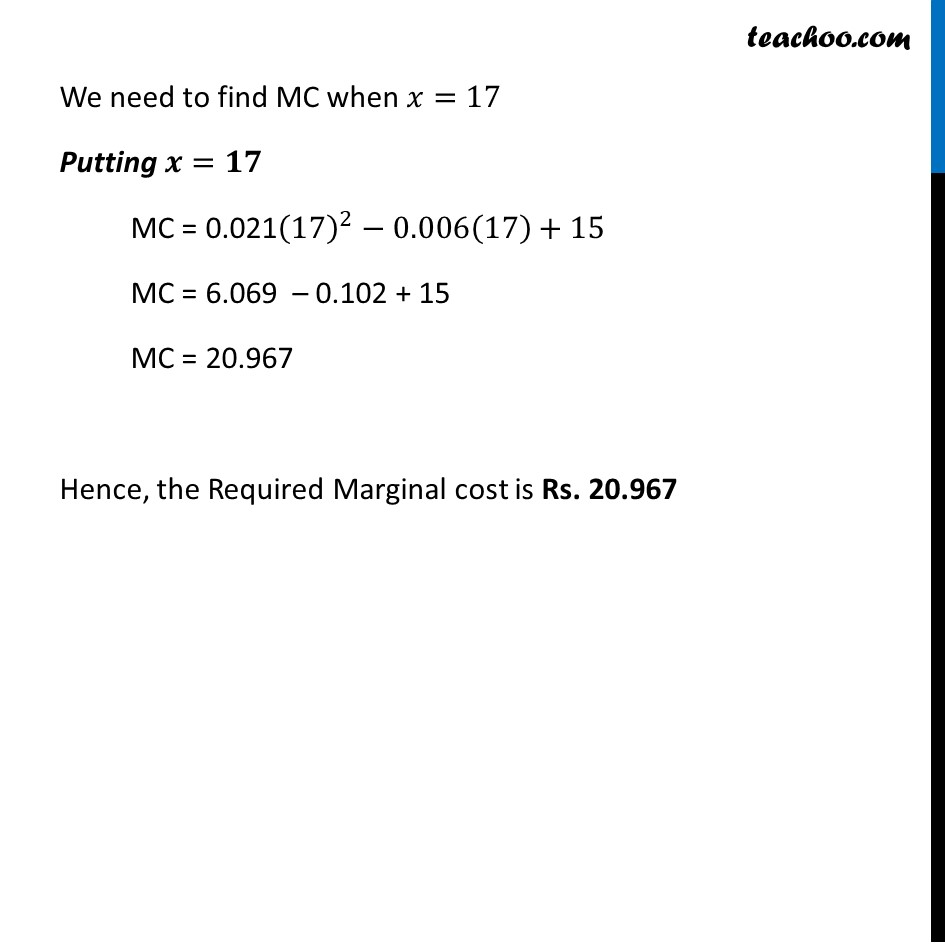1. Chapter 6 Class 12 Application of Derivatives (Term 1)
2. Serial order wise
3. Ex 6.1

Transcript

Ex 6.1, 15 The total cost C(𝑥) in Rupees associated with the production of 𝑥 units of an item is given by 𝐶(𝑥) = 0.007𝑥^3 – 0.003𝑥2 + 15𝑥 + 4000. Find the marginal cost when 17 units are produced.Since Marginal Cost is Rate of change in Total Cost w.r.t No of units produced Let MC be marginal cost So, MC = 𝒅𝑪/𝒅𝒙 Given, Total Cost = C(𝑥) =0.007𝑥^3−0.003𝑥^2+15𝑥+4000" " We need to find marginal Cost when 17 units are produced i.e. MC when 𝑥 = 17 MC = 𝑑(𝐶(𝑥))/𝑑𝑥 MC = 𝑑(0.007𝑥^3 − 0.003𝑥^2 + 15𝑥 + 4000)/𝑑𝑥 MC = 𝑑(0.007𝑥^3 )/𝑑𝑥 − 𝑑(0.003𝑥^2 )/𝑑𝑥+ 𝑑(15𝑥)/𝑑𝑥+ 𝑑(4000)/𝑑𝑥 MC = 0.007 𝑑(𝑥^3 )/𝑑𝑥 −0.003 𝑑(𝑥^2 )/𝑑𝑥 + 15𝑑(𝑥)/𝑑𝑥+0 MC = 0.007 × 3𝑥^2−0.003 ×2𝑥+15 MC = 0.021𝑥^2−0.006𝑥+15 We need to find MC when 𝑥=17 Putting 𝒙=𝟏𝟕 MC = 0.021(17)^2−0.006(17)+15 MC = 6.069 – 0.102 + 15 MC = 20.967 Hence, the Required Marginal cost is Rs. 20.967

Ex 6.1

Chapter 6 Class 12 Application of Derivatives (Term 1)
Serial order wise